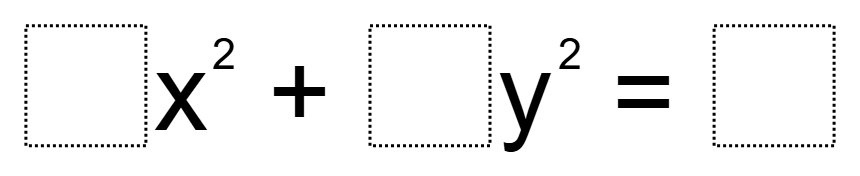# Make the Biggest Circle

Directions: Using the digits 1 to 9 as many times as you want, fill in the boxes to make the biggest circle:### Hint

Where is it better to put the larger values?

### Answer

To make the circle bigger, the radius needs to be as large as possible.  So, putting a 9 there makes it the biggest.  The coefficients of x and y need to be as small as possible so they both need to be 1.

Source: Robert Kaplinsky

## Pythagorean Inequality

Directions: Using the digits 1 through 6 at most one time each, fill in the …